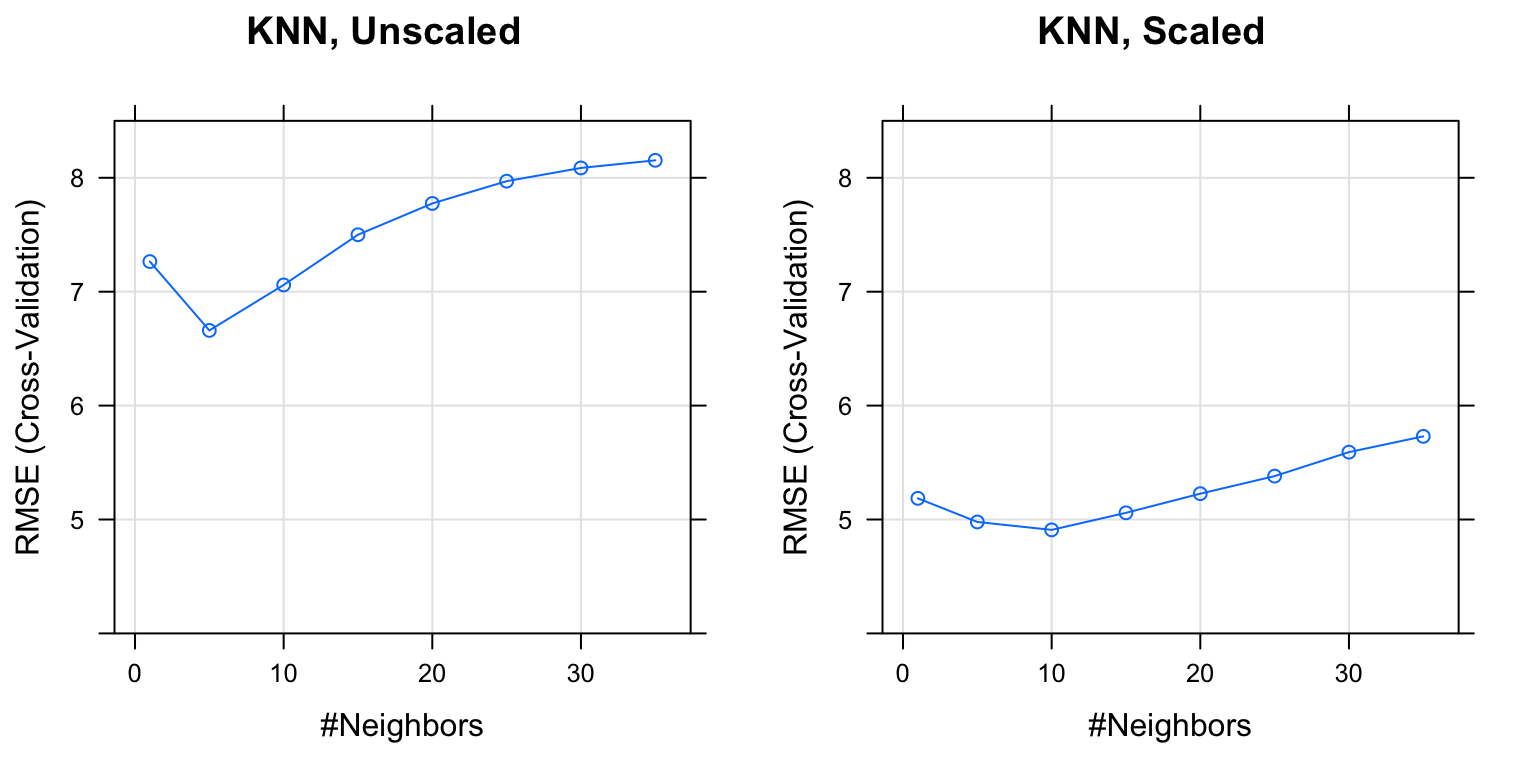For this homework, you may only use the following packages:

# general
library(MASS)
library(caret)
library(tidyverse)
library(knitr)
library(kableExtra)
library(mlbench)

# specific
library(randomForest)
library(gbm)
library(klaR)
library(ellipse)

# Exercise 1 (Tuning KNN Regression with caret)

[6 points] For this exercise we will train KNN regression models for the Boston data from the MASS package. Use medv as the response and all other variables as predictors. Use the test-train split given below. When tuning models and reporting cross-validated error, use 5-fold cross-validation.

data(Boston, package = "MASS")
set.seed(1)
bstn_idx = createDataPartition(Boston\$medv, p = 0.75, list = FALSE)
bstn_trn = Boston[bstn_idx, ]
bstn_tst = Boston[-bstn_idx, ]

Consider $$k \in \{1, 5, 10, 15, 20, 25, 30, 35\}$$ and two pre-processing setups:

• Do not scale the predictors.
• Do scale the predictors.

Provide plots of cross-validated error versus tuning parameters for both KNN pre-processing setups. Use the same value on the $$y$$ axis for both plots. (You can be lazy and let caret create these plots. Since it will use lattice plotting, putting them side-by-side, or on the same plot would be difficult.)

Solution:

set.seed(1337)
bstn_knnu_mod = train(
medv ~ .,
data = bstn_trn,
trControl = trainControl(method = "cv", number = 5),
method = "knn",
tuneGrid = expand.grid(k = c(1, 5, 10, 15, 20, 25, 30, 35))
)
set.seed(1337)
bstn_knns_mod = train(
medv ~ .,
data = bstn_trn,
trControl = trainControl(method = "cv", number = 5),
preProcess = c("center", "scale"),
method = "knn",
tuneGrid = expand.grid(k = c(1, 5, 10, 15, 20, 25, 30, 35))
)# Exercise 2 (More Regression with caret)

[7 points] For this exercise we will train more regression models for the Boston data from the MASS package. Use medv as the response and all other variables as predictors. Use the test-train split given previously. When tuning models and reporting cross-validated error, use 5-fold cross-validation.

Traing a total of three new models:

• A random forest
• Use the default tuning parameters chosen by caret
• A boosted tree model (Use gbm)
• Use the provided tuning grid below
gbm_grid = expand.grid(interaction.depth = c(1, 2, 3),
n.trees = (1:20) * 100,
shrinkage = c(0.1, 0.3),
n.minobsinnode = 20)

Provide plots of error versus tuning parameters for the the boosted tree model. Also provide a table that summarizes the cross-validated and test RMSE for each of the three (tuned) models as well as the two models tuned in the previous exercise.

Solution:

set.seed(1337)
bstn_lm_mod = train(
medv ~ .,
data = bstn_trn,
trControl = trainControl(method = "cv", number = 5),
method = "lm"
)
set.seed(1337)
bstn_rf_mod = train(
medv ~ .,
data = bstn_trn,
trControl = trainControl(method = "cv", number = 5),
method = "rf"
)
set.seed(1337)
bstn_gbm_mod = train(
medv ~ .,
data = bstn_trn,
trControl = trainControl(method = "cv", number = 5),
method = "gbm",
tuneGrid = gbm_grid,
verbose = FALSE
)